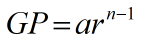Request a Tool

Geometric Progression Calculator

Geometric Progression Calculator is use to calculate geometric progression from nth term, first term and common difference.

Output

Geometric Progression
0

Formula• GP = Geometric Prograssion
• n = nth term
• a = First term
• r = Common diffrance

Defination / Uses

A geometric progression, also known as a geometric sequence, is a non-zero numerical sequence in which each term after the first is determined by multiplying the preceding term by a fixed, non-zero value called the common ratio.

With our geometric sequence calculator, you can calculate anything about a geometric progression. This geometric series calculator will assist you in understanding the definition of a geometric sequence, allowing you to respond to the question, "What is a geometric sequence?" With several intriguing geometric series examples, we show the differences between both geometric sequence equations, the explicit and recursive formula for a geometric sequence, and how to use the geometric sequence formula. We've also created a function called "geometric series calculator" that calculates the sum of a geometric sequence.